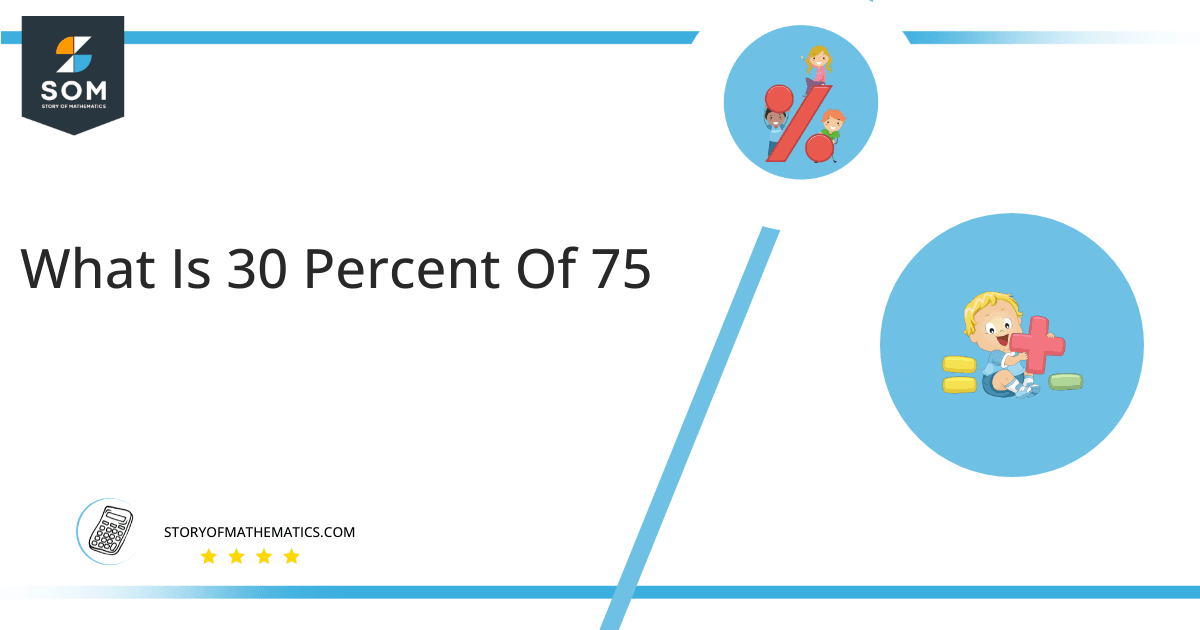# What Is 30 Percent of 75 + Solution with Free Steps?The 30 percent of 75 is equal to 22.5. It can be easily calculated by dividing 30 by 100 and multiplying the answer with 75 to get 22.5.

The easiest way to get this answer is by solving a simple mathematical problem of percentages. You need to find 30% of 75 for some sale or real-life problem. Divide 30 by 100, multiply the answer by 75, and get the 30% of 75 value in seconds.

This article will explain the full process of finding any percentage value from any given quantity or number with easy and simple steps.

## What is 30 percent of 75?

The 30 percent of 75 is 22.5.

The percentage can be understood with a simple explanation. Take 75, and divide it into 100 equal parts. The 30 parts from the total of 100 parts is called 30 percent, which is 22.5 in this example.

## How To Calculate 30 percent of 75?

You can find 30 percent of 75 by some simple mathematical steps explained below.### Step 1

Firstly, depict 30 percent of 75 as a fractional multiple as shown below:

30% x 75

### Step 2

The percentage sign % means percent, equivalent to the fraction of 1/100.

Substituting this value in the above formula:

= (30/100) x 75

### Step 3

Using the algebraic simplification process, we can arithmetically manipulate the above equation as follows:

= (30 x 75) / 100

= 2250 / 100

= 22.5This percentage can be represented on a pie chart for visualization. Let us suppose that the whole pie chart represents the 75 values. Now, we find 30 percent of 75, which is 22.5. The area occupied by the 22.5 value will represent the 30 percent of the total 75 values. The remaining region of the pie chart will represent 70 percent of the total 75 values. The 100% of 75 will cover the whole pie chart as 75 is the total value.

Any given number or quantity can be represented in percentages to better understand the total quantity. The percentage can be considered a quantity that divides any number into hundred equal parts for better representation of large numbers and understanding.

Percentage scaling or normalization is a very simple and convenient method of representing numbers in relative terms. Such notations find wide application in many industrial sectors where the relative proportions are used.